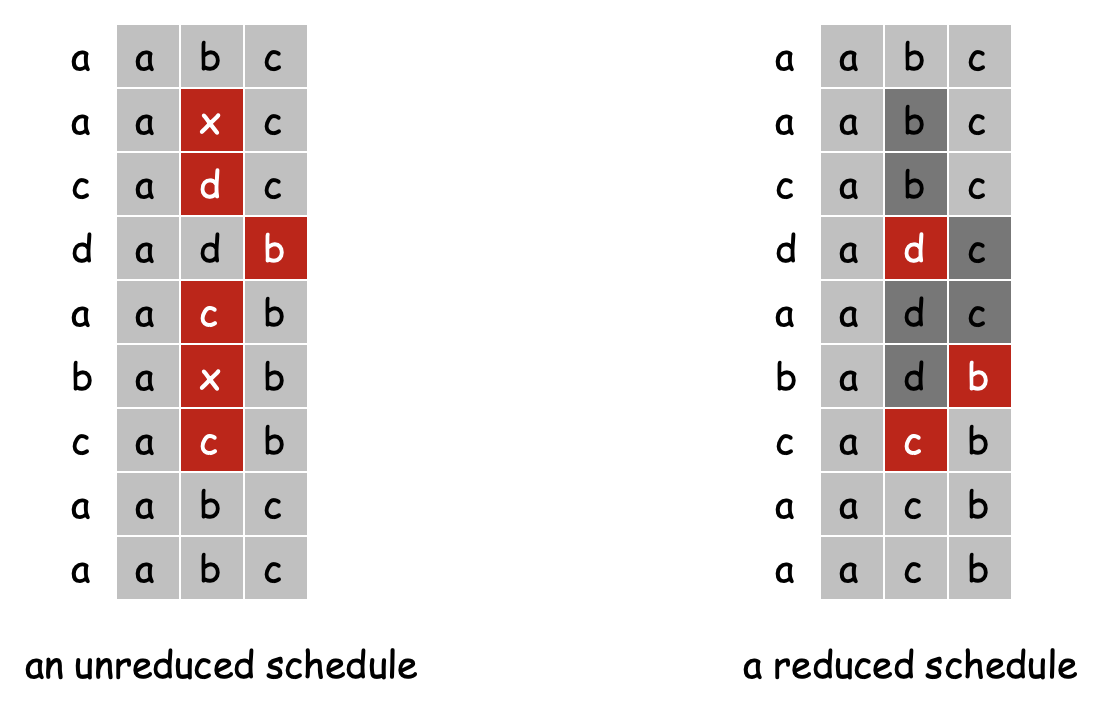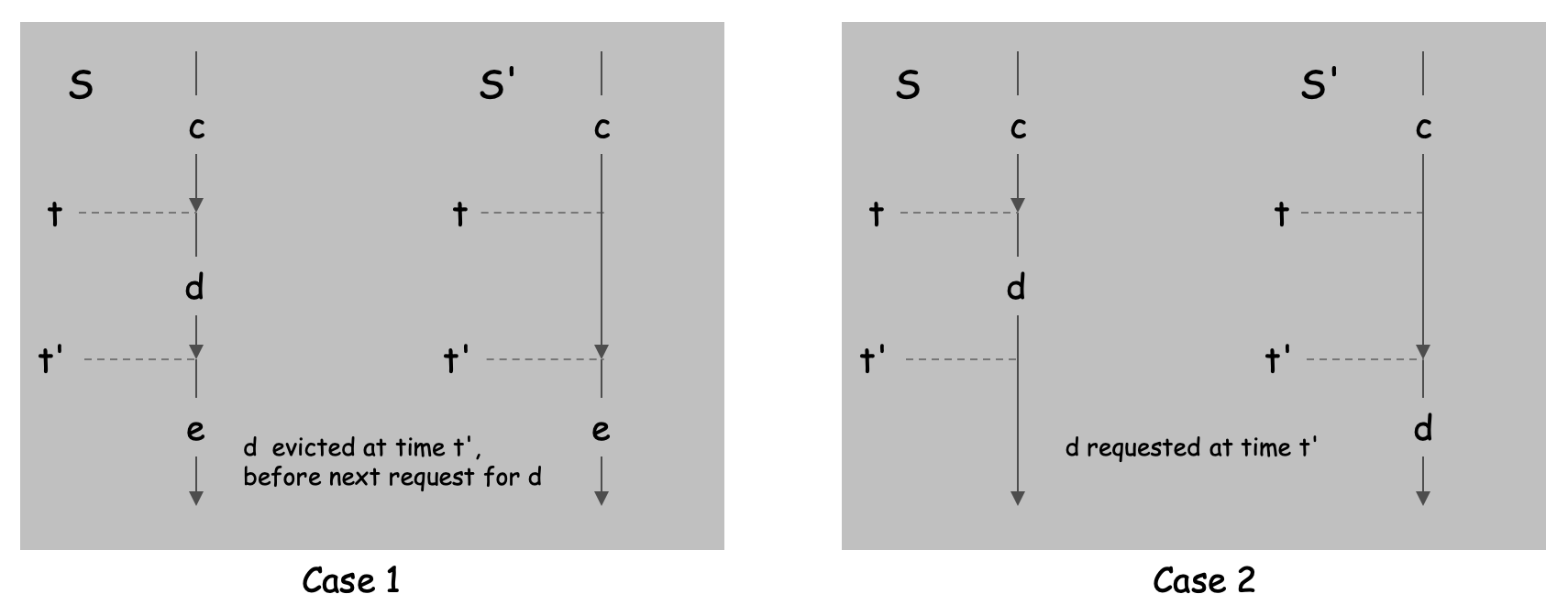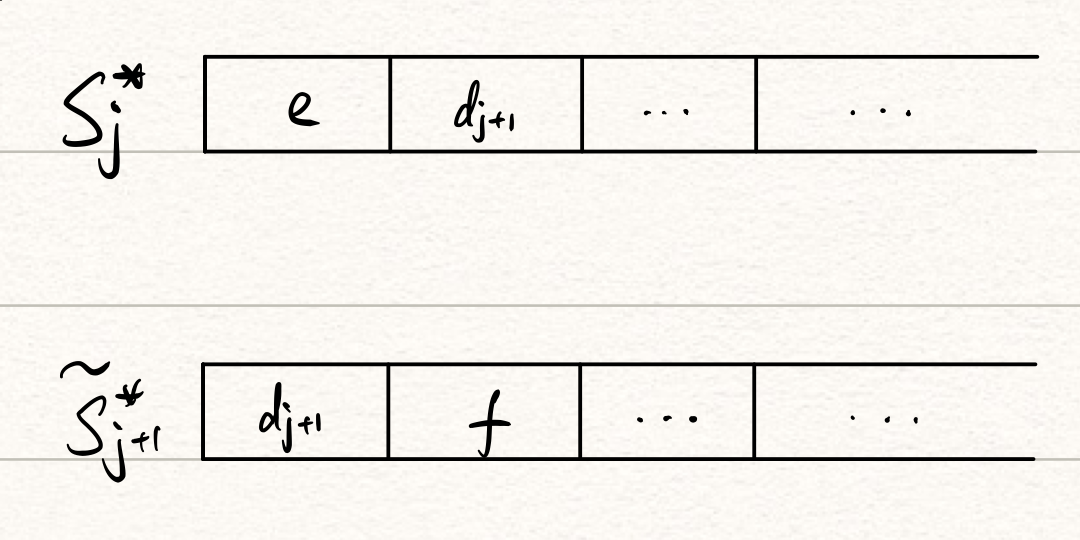# 算法复习笔记：贪心求最优Caching策略

Weijie Huang published on
9 min, 1749 words

Categories: Notes Legacy

## Farthest-in-Future（FF）算法

### 简化调度*：在非简化调度中，请求失败之外的block写入也被视为一次miss（即换页，需要耗费大量时间从内存中读取数据）。

1. 写入$d$后没收到对$d$的请求，而是在$t'$时刻收到了对另外一个不在内存中的block（记作$e$）的请求。此时省去$d$的写入，可减少1次miss。
2. 写入$d$后在$t'$时刻收到了对$d$的请求。此时将写入$d$的操作移至$t'$时刻，miss数不变。### FF算法的最优性

Farthest-in-Future算法得出的策略$S_\text{FF}$是最优策略

• $n=0$时，该命题显然成立。

• $n = j(j \geq 0)$时，假设该命题成立，即存在某最优简化调度$S^*_j$满足前$j$个请求的调度策略与$S_\text{FF}$相同，下面尝试构造使命题成立的$S^*_{j+1}$：

考虑第$j+1$个请求$d_{j+1}$，有以下若干种情况：

1. $d_{j+1}$在缓存中，此时$S^*_{j+1} = S^*_j$不做操作，显然和$S_\text{FF}$的前$j+1$个策略相同。

2. $d_{j+1}$不在缓存中，且$S^*_j$和$S_\text{FF}$替换掉相同的block，则$S^*_{j+1} = S^*_j$和$S_\text{FF}$的前$j+1$个策略显然相同。

3. $d_{j+1}$不在缓存中，$S_\text{FF}$替换掉$e$，$S^*_j$替换掉$f$且$f \neq e$，下面需证明$S_\text{FF}$的策略不比$S^*_j$差

构造$\tilde S^*_{j+1}$使得前$j$个请求的调度与$S^*_j$相同、第$j+1$个请求和$S_\text{FF}$相同，假设$k$时刻（$k > j+1$）$\tilde S^*_{j+1}$和$S^*_j$第一次执行不同操作，对应请求为$d_k$。下图为此时两种策略分别对应的缓存状态（$d_{j+1}$可能被同时替换成其它block）：1. 若$d_k = e$，容易推出此时$f$没有出现。由于$S_\text{FF}$在第$j+1$个请求时替换了$e$，则$e$一定比$f$更晚出现，产生矛盾。
2. 若$d_k = f$，此时$f$不在$S^*_j$对应的内存中，令$e’$为$S^*_j$替换的block：
• 若$e’ = e$，此时$\tilde S^*_{j+1}$直接从缓存中读取$f$，二者缓存内容相同。
• 若$e’ \neq e$，令$\tilde S^*_{j+1}$将$e’$替换成$e$，使二者缓存内容相同。此时$\tilde S^*_{j+1}$不是简化调度，但可以由上述讨论在不增加miss数的前提下转化成简化调度，并且满足前$j+1$步与$S_\text{FF}$相同**。
3. 若$d_k \neq e$且$d_k \neq f$，由于$\tilde S^*_{j+1}$和$S^*_j$执行不同操作，此时$S^*_j$必然替换$e$。令$\tilde S^*_{j+1}$替换$f$，则二者缓存内容再次相同。

综上，可以构造出$S^*_{j+1} = \tilde S^*_{j+1}$使命题在$n = j+1$时成立。

**：人为添加一次替换后，$\tilde S^*_{j+1}$在$k$时刻视为出现一次miss，和$S^*_j$在$k$时刻的请求结果相同；又因为除$k$时刻、$j+1$时刻外二者行为一致，显然二者miss数相等。此外，为了符号表示方便，转化后的简化调度仍然记作$\tilde S^*_{j+1}$。

## 后记

1. 证明贪心结果不比其它算法差；
2. 最优结果的性质，贪心结果同样满足；
3. 能够在不使答案“变差”的前提下将其它答案转化成贪心答案。